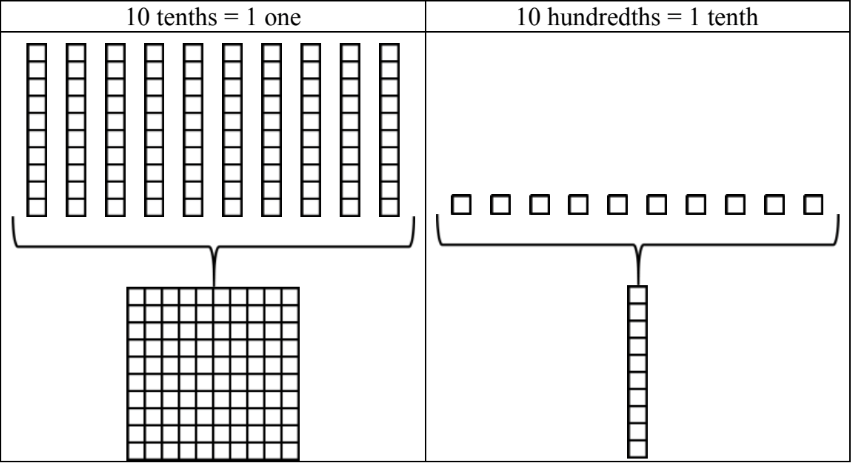#### Everything You Need in One Place

Homework problems? Exam preparation? Trying to grasp a concept or just brushing up the basics? Our extensive help & practice library have got you covered.#### Learn and Practice With Ease

Our proven video lessons ease you through problems quickly, and you get tonnes of friendly practice on questions that trip students up on tests and finals.#### Instant and Unlimited Help

Our personalized learning platform enables you to instantly find the exact walkthrough to your specific type of question. Activate unlimited help now!

0/1
##### Intros
###### Lessons
How to add decimals with base ten (block) models
0/14
##### Examples
###### Lessons
1. Regrouping practice for decimals with block models
Regroup so that each place value only has one digit. Use base ten (block) models to help.
1. 17 tenths = ____ ones + ____ tenths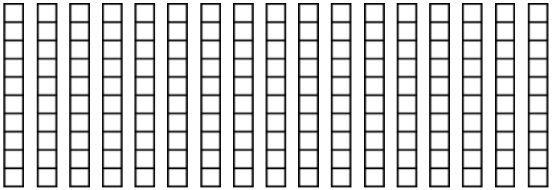2. 4 ones + 12 tenths + 39 hundredths = ____ ones + ____ tenths + ____ hundredths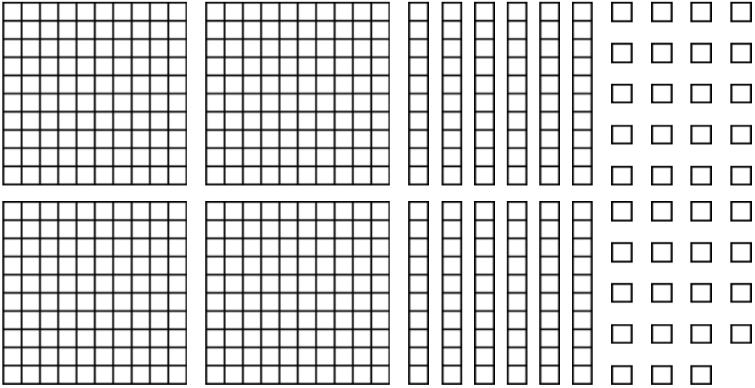3. 6 ones + 29 tenths + 16 hundredths = ____ ones + ____ tenths + ____ hundredths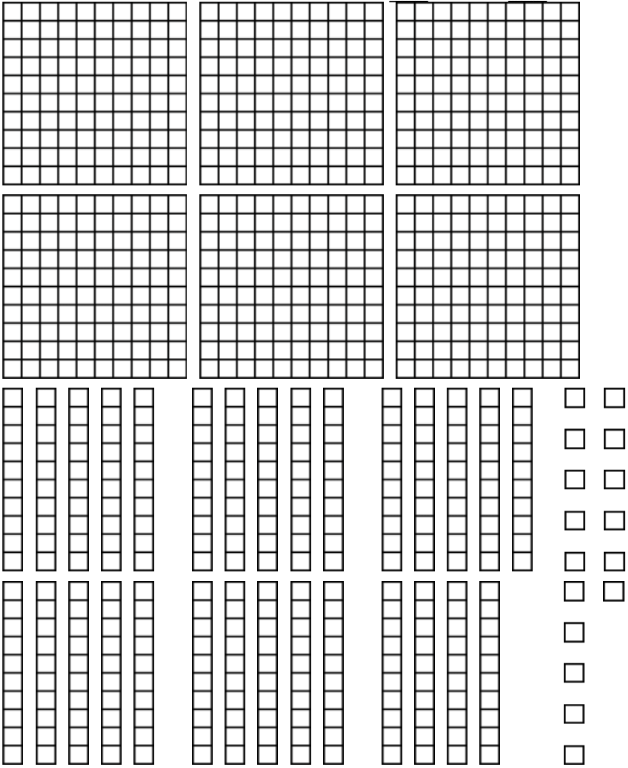1.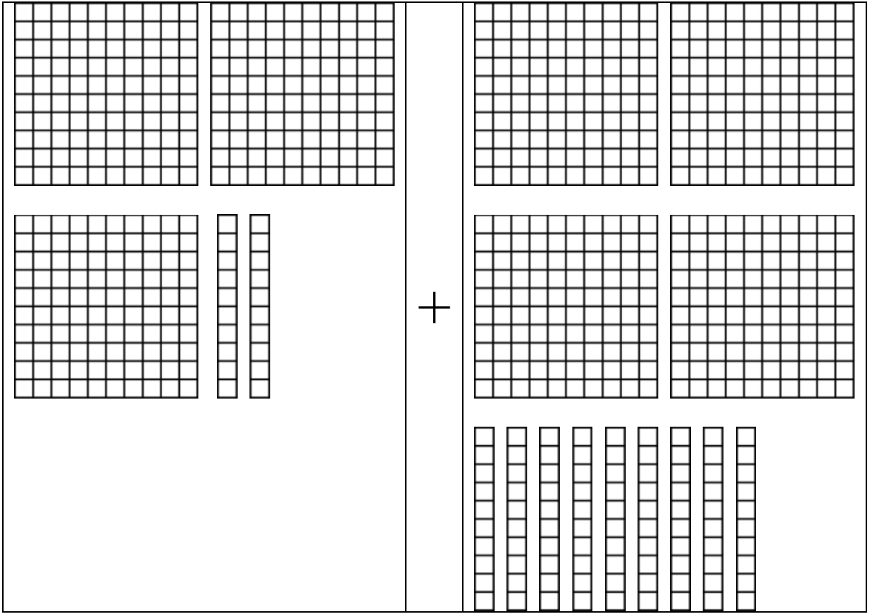2.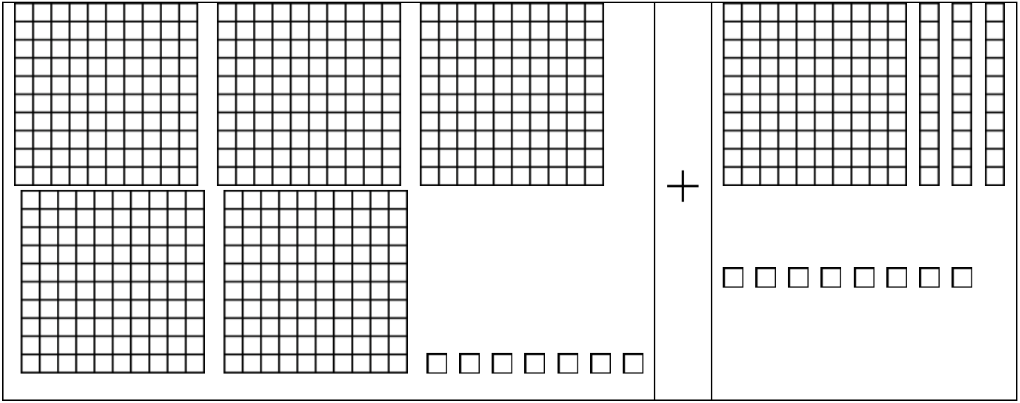3.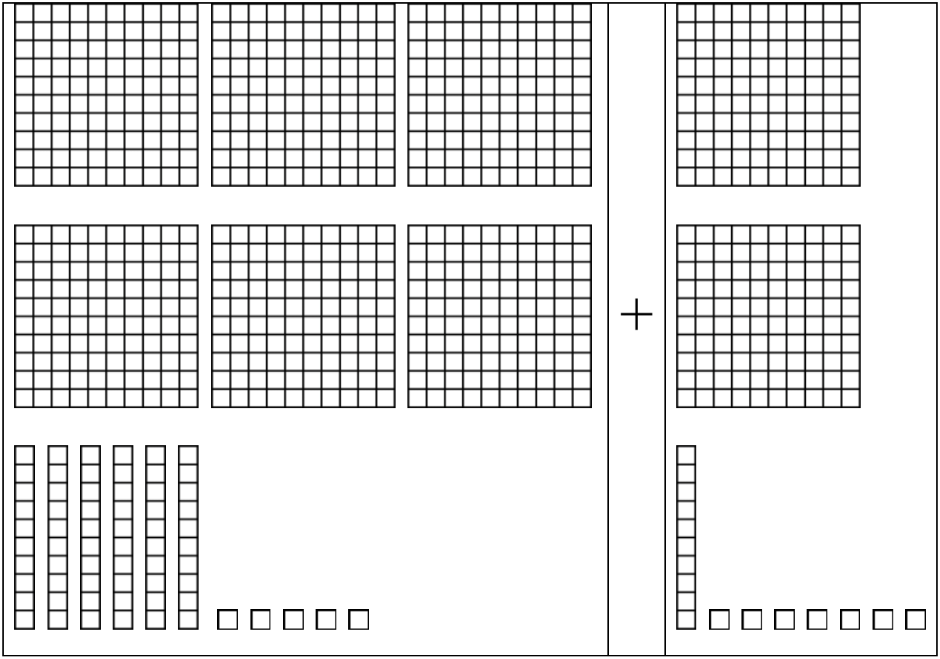3. Adding decimals on a grid
Add the decimals by lining up on the grid. Regroup where necessary.
1. 34.8 + 5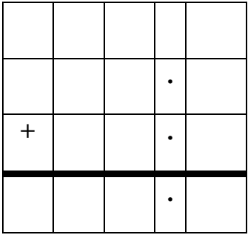2. 4.6 + 2.9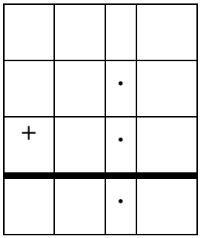3. 41.03 + 5.72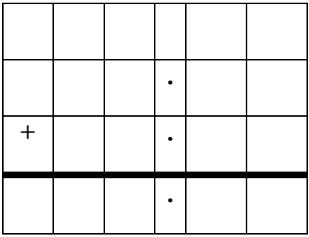4. 35.84 + 6.5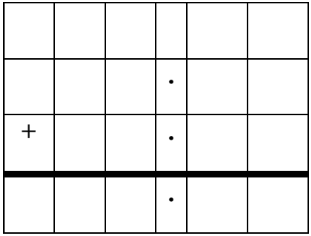4. Adding decimals and rounding decimal sums
1. 7.6 + 2.14
2. 80.65 + 19.23
3. 18.24 + 3.17
4. 98 + 38.97
0%
##### Practice
###### Topic Notes

In this lesson, we will learn:

• How to add decimal numbers using base ten (block) models
• That adding decimal numbers is the same as adding whole numbers, which involves lining up the right place values and regrouping

Notes:

• To add decimals with base ten (block) models:
• Remember the three types of blocks used to represent the ones, tenths, and hundredths place values
• "One whole" or hundred block = ones place value
• Column (stick or rod) = tenths place value
• Single square = hundredths place value